metapost

This is an old revision of the document!

…is code to define what the labels and cave symbols in pdf and svg outputs look like.

pstoedit translates PostScript and PDF graphics into other vector formats, Metapost format too.
TUG's listing of MetaPost on the Web
MetaPost tutorial, Robert Špalek, česky
Many interesting MetaPost examples, gallery what is possible to do with MP
Page with many links to Metapost documentation and examples
Learning METAPOST by Doing, André Heck (pdf)
Loria: The Metafun manual, Hans Hagen, 2017 (pdf)
or: Pragma: The Metafun manual, Hans Hagen, 2017 (pdf)
Puzzling graphics in METAPOST, Hans Hagen (pdf)
Many nice examples
Many links from Michio Matsuyama, but you have to copy the text and open it as html file

If you are brave Marco has written more than I ever wanted to know here Therion by Examples Metapost and here - Chapter 7

1. Create a new symbol with your favorite vector graphics editor,
2. Save results to .eps format, and then
3. Use pstoedit to convert the .eps to metapost,
4. Adapt the code to work with Therion (Refer to section 7.4 of Marco Corvi's Therion by Examples), then

A limitation is that pstoedit converts only to polylines. It does not create 'curves' or other more sophisticated Metapost entities. It is enough to create user symbol for a tree for example.
Another way is to draw your symbol on graph paper and then create Metapost code for straight or smooth curved lines.
Both approaches will in due course require you to learn some Metapost!
You may check the very basic tutorial of Metapost here:http://meeting.contextgarden.net/2008/talks/2008-08-22-hartmut-metapost/mptut-context2008.pdf

#### Implementing redefined metapost examples (Bruce Mutton)

These examples show how redefined symbols like those below can be used, and includes a redefinition of Atlas so that it includes a north arrow on each page, and a redefinition of the continuation symbol so that it displays the associated text.

You can use this code as a starting point to make your own customisations.
You can either start with the unadulterated source code (perhaps the best way, and as described here) or you can use the code that has been updated with any modifications already present in your current setup (often useful as well if you want to see the end result of your definitions and re-definitions)- as described below.

You need to run Therion in debug mode (command line -d option)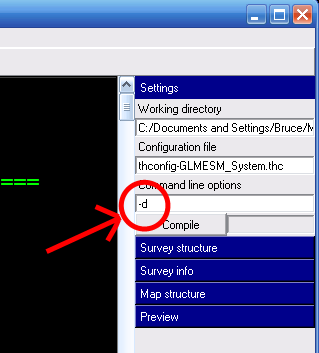to tell it to produce and retain a folder with interim outputs (not to be confused with layout-debug which can produce 2D outputs with station names, scrap names, intermediate scrap distortions etc).

The folder produced is called thTMPDIR

It contains;

• data.mp = a dump of all the metapost code used by Therion
• data.tex = a dump of all the tex code used by Therion

and perhaps less usefully…

• th_texts.tex = ascii dump of all tex variables
• th_legend.tex = similar dump of the legend
• and much more…

Skim through data.mp and data.tex to find definitions and snippets of code that you'd like to edit.

Copy these to a code-tex or code-metapost block in a layout in one of your *.th or thconfig-*.* files, and make your edits.

The files produced in thTMPDIR will now reflect the code you have entered in your layouts.

By the way, you might be able to use the following to track down errors in metapost code (or in scrap drawings).

On Sun, Feb 7, 2010 Bruce wrote:

Is u (and v w for that matter) intended to correspond to a
particular drawn entity size in the finished pdf, or are they just
arbitrary variables for which one can calculate the value of, using
the code in the initialise definition?
Bruce

u allows nonlinear scaling of symbols depending on scale. The macro initialize assigns a fixed value to 'u' which will be used in pdf for that scale. On average, diameter of point symbols is (or is intended to be) 1u in the output.

v and w behave differently (v could be used e.g. for some area fills with decreasing distance among symbols for smaller scales but only up to a certain threshold, then bigger distance again; w is almost constant). Currently they are not (or perhaps only occasionally) used in symbols design.

In the beginning we wanted to do some sort of optimization of values assigned to u, v, w but did not manage to do it so far. It would be useful if somebody could test alternative values (and alternative breaks for map scale in the initialize macro) for various scales and various styles of map drawing (lot of symbols vs. sparsely filled map). Good setup of these values could improve map appearance substantially.

Cheers, Martin

Refer to the section New Map Symbols, Point Symbols in The Therion Book.

If you want your custom point symbol to be able to be aligned, rotated or scaled by users according to options set in th2 drawing files, then your T:= identity… line will need include those keywords and the variables parsed in the symbol def statement. A number of the point symbol examples below do not, possibly intentionally preventing those symbols from being aligned, rotated or scaled respectively.

• Symbol coordinates (0u, 0u) are the origin point for that symbol. This is the place in the symbol drawing that corresponds exactly to the point symbol location that you will draw in a scrap with XTherion.
• Your symbols should generally be defined so that they fit within the size range -0.5u to +0.5u in both x and y axes, although you can use larger or smaller values in either dimension. This is because we generally want the map symbols to be about ‘u’ in size.

This image is a symbol that has been defined with maximum x and y coordinates, supposedly, of 0.7u, both positive and negative. The insertion point is at the intersection of the cross hairs, and the pdf has been exported with a rotate value of 15 degrees. It has been overlaid with some code that highlights the origin and alignment loci, and unit symbol size, u, as described below.

The symbol alignment option relies on the definition of a U: variable, otherwise your symbol will cause an error and stop Therion if a user sets an alignment option other than centre. The U: variable is depicted by the light blue box.

• U: is a scaling factor for alignment parameters, such as right, top or top-right.

U: defines the size of the x horizontal (left-right) and y vertical (up-down) offset when a symbol is aligned, so the x and y components of U: should be set to be around the maximum absolute value of x and y symbol coordinates, respectively. ie for a symmetrically placed symbol who’s perimeter will just touch the insertion point, set U:=(0.5x symbol width, 0.5x symbol height).

• If a U: component is smaller than a maximum corresponding symbol coordinate, an aligned symbol will overlap the insertion point.
• If a U: component is larger than a maximum corresponding symbol coordinate, an aligned symbol will have a gap from the insertion point.
• If your symbol has its greatest dimension on a diagonal, then you should increase the U: components accordingly, to avoid unintentional drawing overlaps for aligned symbols.

As one 'use case' for aligning symbols is to enable them to be placed ‘near’ to another symbol, and be always positioned appropriately at a variety of output scales, I am going to suggest that U: components for user friendly point symbols should always be just a little greater than half the symbol dimension. ie the light blue box should enclose the symbol. That way, an aligned symbol can always leave a pleasing small gap from its insertion point.

When writing a new point symbol and testing that it aligns and rotates nicely, it can be a bit tricky to figure out where the origin, insertion point, and alignment loci are located. So I have written some code that you can temporarily place inside a symbol, to get a visual representation of how well placed its origin and alignment loci are with respect to its insertion point, and of its size compared to the nominal default size of u.

Here it is inserted into an electric light symbol definition. The code is between the lines with % % %. It creates a green insertion point, a red origin point that represents the (0u, 0u) origin of the symbol, a light blue rectangle that represents the loci of the alignment options (left, top-left etc), and a grey unit symbol size. Note that this code requires that T:= … rotated BEFORE aligned. If your symbol uses T:= … aligned BEFORE rotated, then the code needs to be modified slightly.

After writing the code, I realised that this symbol was in fact larger than 0.7u in the y direction, therefore the U: variable is not tall enough, the symbol is not centered around around its (0u,0u) coordinates (which might be OK if you want the space below the luminaire to be part of the 'symbol') and it is just a bit (but probably acceptably) larger than u in size!

   def p_u_electriclight (expr pos,theta,sc,al) =
U:=(0.7u, 0.7u);
% Reduce size of the symbol.  The default size is too big.
interim defaultscale:=0.4sc;
T:=identity rotated theta aligned al scaled defaultscale shifted pos; %corrected to rotate THEN align

% % %  SYMBOL PARAMETER INDICATOR (origin, insertion, alignment box, u box)
% Placing this code directly after T:= identity line will put the indicators under the symbol
% Placing this code immediately before enddef will put the indicators over top of the symbol
% Before use you need to correctly assign the parsed variable aliases in the next two rows from the def statement above
rotation:= theta;               %set this to the third parsed variable (ie theta)
pair alignment; alignment:= al; %set this to the last parsed variable (ie al)

% show symbol origin, (0,0) red
thdraw fullcircle scaled 0.15u withpen PenD withcolor red;
% show symbol insertion point, (0,0) green
thdraw fullcircle shifted -(xpart alignment * xpart U, ypart alignment * ypart U) rotated -rotation withpen PenD withcolor green;
% show U alignment box light blue
q:= (xpart U, -ypart U) -- (xpart U, ypart U) -- (-xpart U, ypart U) -- (-xpart U, -ypart U) -- cycle;
thdraw q shifted -(xpart alignment * xpart U, ypart alignment * ypart U) rotated -rotation withpen PenD withcolor 0.5blue+0.5white;
%	show u box grey
thdraw unitsquare scaled u shifted (-0.5u, -0.5u) withpen PenD withcolor 0.1black+0.5white;
% % %

pickup PenC;
thdraw fullcircle scaled 0.5u shifted (0.0u, 0.7u);
thdraw (-0.5u, -0.6u) -- (-0.5u, 0.0u);
thdraw (-0.5u, 0.0u) .. (-0.35u, 0.55u) .. (0.0u, 0.7u);
thdraw (0.0u, 0.7u) .. (0.35u, 0.55u) .. (0.5u, 0.0u);
thdraw (0.5u,0.0u) -- (0.5u, -0.6u);
thdraw (-0.7u, -0.6u) -- (0.7u, -0.6u);
enddef;

Here is an example with the above point symbol that has been redefined with three different U: settings, and inserted with -align top-right. On the left, U:=(1.0u, 1.0u) [U/u=1.4], in the centre, U:=(0.7u, 0.7u) [U/u=1.0], on the right, U:=(0.5u, 0.5u) [U/u= 0.7]. Note that the alignment, top-right, is relative to the local coordinate system in the scrap, not necessarily to the output page coordinate system. In these examples it does match the output page orientation, because the scrap has 'north up'.

The smudgy line is intended to represent an object that the alignment is intended to avoid, ie a wall. You can see that U: = symbol dimension is OK, but U:= smaller than symbol dimension is probably what most users would want to avoid.

I'm going to suggest that the best values for U: components are between [U/u=1.0] to [U/u=1.2]

Bruce

This can be used to set a minimum pen thickness, so that all other pens take their sizes relative to that pen thickness. This can be useful for publications that mandate a specific pen width, which can be harder to control with scaling. (This can be done by setting the “u” value, but that causes all symbols to be scaled too.)

code metapost
def minimumpen = 0.18pt enddef;
def PenA = pencircle scaled (minimumpen*1/0.35) enddef;
def PenB = pencircle scaled (minimumpen*0.7/0.35) enddef;
def PenC = pencircle scaled (minimumpen*0.5/0.35) enddef;
def PenD = pencircle scaled (minimumpen) enddef;
def PenX = pencircle scaled (minimumpen*1.2/0.35) enddef;
endcode

For example; centrelines, water, point altitudes

#### Define a user point symbol for a stalagmite boss

def p_u_boss (expr pos,theta,sc,al)=
T:=identity aligned al rotated theta scaled sc shifted pos;
pickup PenD;
p := (0.08u,0.25u)..(0,0.29u)..(-0.08u,0.25u);
q := (0.16u,0.5u)..(0u,0.58u)..(-0.16u,0.5u);
for i=0 upto 9:
thdraw p rotated 36i;
thdraw q rotated 36i;
endfor
p := fullcircle scaled 0.15u;
thdraw p;
enddef;

#### Define an entrance symbol as a theta inside a diamond

def p_entrance_MY (expr pos,theta,sc,al)=
U:=(.2u,.5u);
T:=identity aligned al rotated theta scaled sc shifted pos;
path p;
p = (-.3u,-.25u) -- (-.2u,-.25u){dir 135} .. (0u, .25u) .. {dir 225}(.2u,-.25u) -- (.3u,-.25u);
thdraw p withpen PenA;
thdraw unitsquare scaled u shifted (-0.5u,-0.5u) rotated 45 withpen PenD;
enddef;

#### Define a user point symbol for a single large irregular block

def p_u_block(expr pos,theta,sc,al) =
T:=identity aligned al rotated theta scaled sc shifted pos;
path p q;
p := (2.3u,0.9u)--(0.65u,1u)--(-0.9u,0.6u)--(-2.15u,-0.1u)--(-2.35u,-0.25u)--(-2.5u,-0.5u)--(-2u,-0.65u)--(-0.75u,-0.65u)--(0.6u,-0.7u)--(1.1u,-0.5u)--(2.1u,-0.15u)--cycle;
pickup PenB;
thdraw p;
% The following line uses the code from Colour Dependant Visualization of Symbols by Bruce Mutton
if known colour_block_bg: thfill p withcolor colour_block_bg; else: thfill p withcolor (0.75,0.75,0.75); fi;
q := (-2.5u,-0.5u)--(-2u,-0.65u)--(-0.75u,-0.65u)--(0.6u,-0.7u)--(1.1u,-0.5u)--(2.1u,-0.15u)--(2.3u,0.9u)--(2.5u,0.7u)--(2.5u,0.5u)--(2.25u,-0.9u)--(1.1u,-1.3u)--(0.5u,-1.5u)--(-0.75u,-1.4u)--(-2u,-1.15u)--(-2.35u,-0.65u)--cycle;
thdraw q;
thfill q withcolor(0.6,0.6,0.6);
pickup PenD;
path p;
p := (-2u,-0.65u)--(-1.9u,-1u);
thdraw p;
path p;
p := (0.6u,-0.7u)--(0.5u,-1.3u);
thdraw p;
path p;
p := (2.1u,-0.15u)--(2.3u,-0.4u);
thdraw p;
enddef;
initsymbol("p_u_block");

#### Point - Artificial Electric Light

Bill Gee

# This code defines an artificial electric light.  Used in tourist sections of a cave.
# by Bill Gee May 2019
def p_u_electriclight (expr pos,theta,sc,al) =
U:=(0.3u, 0.3u);
% Reduce size of the symbol.  The default size is too big.
interim defaultscale:=0.4sc;
T:=identity aligned al rotated theta scaled defaultscale shifted pos;
pickup PenC;

thdraw fullcircle scaled 0.5u shifted (0.0u, 0.7u);
thdraw (-0.5u, -0.6u) -- (-0.5u, 0.0u);
thdraw (-0.5u, 0.0u) .. (-0.35u, 0.55u) .. (0.0u, 0.7u);
thdraw (0.0u, 0.7u) .. (0.35u, 0.55u) .. (0.5u, 0.0u);
thdraw (0.5u,0.0u) -- (0.5u, -0.6u);
thdraw (-0.7u, -0.6u) -- (0.7u, -0.6u);

enddef;

—-

#### Shell limestone

Point symbol for shell limestone:

def p_u_shell (expr pos,theta,sc,al)=
T:=identity shifted pos;
pickup PenB;
path ss, cesta;
pair za, zb;
turns = 1.55;
za = ( xpart(origin)+0, ypart(origin)+.1u ) rotated 370 turns;
zb = ( xpart(origin)+.3u, ypart(origin)+0 ) rotated 360 turns;
cesta := za--zb;
ss := (origin for t=1 upto 360 turns: -- dir t scaled t endfor) scaled (radius/turns/360);
thdraw ss withcolor (0.3);
thdraw (cesta cutbefore ss) withcolor (0.3);
enddef;

#### P-hangers that face the right way

The default “anchor” symbol takes up a lot of space. It also resists rotation. This produces a P-hanger icon instead. It respects rotation, so that if the “orientation” arrow points to the right, the P-hanger will point in the chosen direction. In addition, it selects the correct direction to face, so that the loop of the hanger always points down the page. It intentionally has a thinner line pointing into the rock, so that it appears to pierce the wall of the cave (just like a real P-hanger).

code metapost
% a P-hanger
def p_anchor_MY (expr P,R,S,A)=
U:=(.25u,.4u);
T:=identity aligned A rotated R scaled S shifted P;
% thick part sticking out of the rock
thdraw (0,0)--(0,.4u) withpen PenB withcolor black;
% thin part in the rock
thdraw (0,-.2u)--(0,0) withpen PenC withcolor black;
% the loop should hang down the "page", based on the rotation
if (R > 0) and (R < 180):
Facing:=-1
else:
Facing:=1
fi;
% stubs to hold the semicircle
thdraw (0,.1u)--(Facing*.1u,.1u) withpen PenB withcolor black;
thdraw (0,.4u)--(Facing*.1u,.4u) withpen PenB withcolor black;
% semicircle
thdraw halfcircle scaled .3u rotated (Facing*-90) shifted (Facing*.1u,.25u) withpen PenB withcolor black;
enddef;
initsymbol("p_anchor_MY");
endcode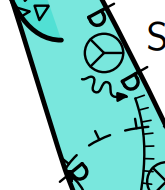Use it with this line in your layout:

symbol-assign point anchor MY

#### Warning triangle

Warning triangle with exclamation mark, and yellow background:

code metapost
% a warning triangle
def p_u_warning (expr P,R,S,A)=
scale:=0.5u;
sin120:=sind(120);
cos120:=cosd(120);
U:=(sin120*scale,scale);
T:=identity aligned A rotated R scaled S shifted P;
% yellow triangle
thfill (0,scale)--(sin120*scale,cos120*scale)--(sind(240)*scale,cosd(240)*scale)--cycle withcolor (1,1,0);
% black triangle outline
thdraw (0,scale)--(sin120*scale,cos120*scale) withpen PenB withcolor black;
thdraw (sin120*scale,cos120*scale)--(sind(240)*scale,cosd(240)*scale) withpen PenB withcolor black;
thdraw (sind(240)*scale,cosd(240)*scale)--(0,scale) withpen PenB withcolor black;
% line of !
thdraw (0,0)--(0,.5*scale) withpen PenB withcolor black;
thdraw (0,-.25*scale)--(0,-.25*scale) withpen PenB withcolor black;
% dot of !
enddef;
initsymbol("p_u_warning");
endcode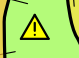Select this as point type “u” with “-subtype warning” in its options. Use it with this line in your layout:

text en "point u:warning" "warning/danger"


#### View whole centerline for underground

Stacho Mudrak

If you wish to view whole centerline for underground, just redefine symbol in layout:

    code mpost
let l_survey_cave = l_survey_surface_SKBB;
endcode

—-

#### Green colored continuous centerline

from Thomas Holder for version 5.2.x…

  def l_survey_cave (expr p) =
pickup PenC;
draw p withcolor 0.5green;
enddef;

#### Make unsurveyed wall lines light-weight and dashed

A sample of how it looks is in the 'line rope' example below.

  code metapost
%Bruce Mutton 2010.06.20 for Therion 5.3.9
def l_wall_unsurveyed (expr P) =
T:=identity;
pickup PenC;
thdraw P dashed evenly scaled (2*optical_zoom);
enddef;
endcode

—-

#### Visualize cave centreline shot flags with colour for splay and duplicate, and dash for approximate shot flags

For version 5.3.12+ Dec2013 (Bruce Mutton)

code metapost #thin grey cave splays, yellow duplicates and dashed approximate legs
def l_survey_cave (expr P) =
% always draws full centreline, rather than short stubs like default cave centrelines
T:=identity;
pickup PenC;
if ATTR__shotflag_splay:
drawoptions(withcolor(0.5,0.5,0.5) withpen PenD);
thdraw P; %  grey & thin
drawoptions();
else: % not splay but may have either or both duplicate and approx flags set
if ATTR__shotflag_duplicate:
drawoptions(withcolor (1,1,0)); % differentiate duplicate with colour yellow
fi;

if ATTR__shotflag_approx:
thdraw P dashed evenly scaled optical_zoom;	% differentiate approx with dashed
else:
thdraw P;
fi;
thdrawoptions();
fi;
enddef;
endcode


I was trying to get the duplicate and approximate symbols to appear in the legend as below, but so far it does not work…

initsymbol("l_survey_cave_duplicate");  %not working

def l_survey_cave_duplicate_legend =
l_survey_cave_duplicate(((.2,.2) -- (.8,.8)); inscale) %not working
enddef;


—-

#### Define NZSS line wall presumed

Draws dashes with v shaped tick marks outside the passage like we tend to do in New Zealand…
A sample of how it looks is in the 'line rope' example below.

  code metapost
%Bruce Mutton 2010.06.20 uses general code and adjust_step defined in therion source code by Martin Budaj
%for Therion 5.3.8
def l_wall_presumed (expr P) =
T:=identity;
cas := 0;                                 % cursor to step along path
dlzka := arclength P;
mojkrok:=adjust_step(dlzka, 1.5u);        % symbol length nudged to be multiple of path length
q  := (-0.2u,-0.4u)--(0,0)--(0.2u,-0.4u); % define v shape
forever:
t1 := arctime (cas + mojkrok*1/5) of P;
t  := arctime (cas + mojkrok/2) of P;
t2 := arctime (cas + mojkrok*4/5) of P;
pickup PenA;                          % thick
thdraw (subpath (t1,t2) of P);          % dash
pickup PenC;                          % thin
thdraw q rotated angle(thdir(P,t)) shifted (point t of P ); % v shape

cas := cas + mojkrok;
exitif cas > dlzka - (2*mojkrok/3);     % for rounding errors
endfor;
enddef;
endcode 

#### Define NZSS line chimney (aven)

  code metapost
%Bruce Mutton 2012.06.10 uses general code for l_pit_UIS defined in therion source code by Martin Budaj 5.3.9
% dots on righthand (rock) side of line spaced 0.2u, 0.2u same as floor-step ticks
def l_chimney (expr P) =
T:=identity;
cas := 0;                            % cursor to step along path
dlzka := arclength P;
mojkrok:=adjust_step(dlzka, 0.25u);  % symbol length nudged to be multiple of path length
q:= (0.20u,-0.20u) -- (0.21u,-0.21u);         % dot

pickup PenC;  %2nd thinnest pen
forever:
t := arctime cas of P;
thdraw q rotated angle(thdir(P,t)) shifted (point t of P ); % draw dots
cas := cas + mojkrok;
exitif cas > dlzka + (mojkrok / 3); % for rounding errors
endfor;
pickup PenB;   %2nd thickest pen
thdraw P;      %continuous line
enddef;
endcode


#### Define NZSS line ceiling-step

This is just Therions default SKBB ceiling step, reflected so that it matches the UIS convention with the tick marks pointing away from the void space.

code metapost
%Bruce Mutton 2012.06.16 uses general code for l_ceilingstep_SKBB defined in therion source code by Martin Budaj 5.3.9
% but ticks on righthand (rock) side of line
def l_ceilingstep (expr P) =
T:=identity;
cas := 0;                            % cursor to step along path
dlzka := arclength P;
mojkrok:=adjust_step(dlzka, 0.8u);   % symbol length nudged to be multiple of path length
pickup PenC;
forever:
t1 := arctime (cas + mojkrok*1/5) of P;
t  := arctime (cas + mojkrok/2) of P;
t2 := arctime (cas + mojkrok*4/5) of P;
thdraw (subpath (t1,t2) of P);
mark_ (P,t,-0.2u);                  % change sign to -0.2u
cas := cas + mojkrok;
exitif cas > dlzka - (2*mojkrok/3); % for rounding errors
endfor;
enddef;
endcode

#### Define NZSS line ceiling-meander

This is just Therions default SKBB ceiling meander, with the symbols reflected so that it matches the UIS convention with the tick marks pointing away from the void space.

code metapost
%Bruce Mutton 2012.06.16 uses general code for l_ceilingmeander_SKBB defined in therion source code by Martin Budaj 5.3.9
% but ticks on outside (rock) side of lines
def l_ceilingmeander (expr P) =
pair Pp;
pair Pd;
pair Pv;
T:=identity;
cas := 0;                           % cursor to step along path
dlzka := arclength P;
mojkrok:=adjust_step(dlzka, 0.8u);  % symbol length nudged to be multiple of path length
pickup PenC;
forever:
t  := arctime (cas + mojkrok/2) of P;
Pp := (point t of P);
Pd := unitvector(thdir(P,t));
Pv := Pd rotated 90;
thdraw (Pp + 0.2u * Pv) --
(Pp + 0.3u * Pv);              % add 0.1u to each moves ticks outside
thdraw (Pp + 0.2u * Pv + 0.2u * Pd) --
(Pp + 0.2u * Pv - 0.2u * Pd);
thdraw (Pp - 0.2u * Pv) --
(Pp - 0.3u * Pv);              % subtract 0.1u to each moves ticks outside
thdraw (Pp - 0.2u * Pv + 0.2u * Pd) --
(Pp - 0.2u * Pv - 0.2u * Pd);
cas := cas + mojkrok;
exitif cas > dlzka - (2*mojkrok/3);  % for rounding errors
endfor;
enddef;
endcode


#### Draw a plank walk and (rope) handrail

  def l_u_plankwalk  (expr P) = T:=identity;
cas := 0;
dlzka := arclength P;
pickup PenD;
forever:
t := arctime cas of P;
thdraw ((point t of P) + 0.5 * u * unitvector(thdir(P,t) rotated 90)) --
((point t of P) - 0.5 * u * unitvector(thdir(P,t) rotated 90) );
cas := cas + mojrok;
exitif cas > dlzka + (mojrok/3);  % for rounding errors
endfor;
pickup PenC;
%thdraw P;
%draw path withcolor (0.5, 0 ,0)
enddef;
   def l_u_handrail (expr P) = T:=identity;
pair zz[];
for t = 0 upto length P - 1:
zz1 := point t of P;
zz2 := point t+1 of P;
zz3 := 0.5[zz1,zz2];
zz4 := 0.25[zz1,zz2];
zz5 := 0.75[zz1,zz2];
pickup PenA;
thdraw zz1 -- zz2 withcolor(.67,.41,.28); %planks
thdraw zz1 shifted (0,1.6u) .. zz4 shifted (0,1.2u) .. zz3 shifted (0,1.6u) .. zz5 shifted (0,1.3u) .. zz2 shifted (0,1.6u) withcolor(.67,.41,.28);
pickup pencircle scaled 1pt;
thdraw zz1 shifted (0,-1.2u) -- zz1 shifted (0,2u) withcolor(.67,.41,.28); %first post
thdraw zz3 -- zz3 shifted (0,2u) withcolor(.67,.41,.28); %mid post
endfor;
thdraw zz2 shifted (0,-6u) -- zz2 shifted (0,2u) withcolor(.67,.41,.30); %last post
enddef;

#### Define a doline

  def l_u_doline (expr P) =
T:=identity;
laenge:= arclength P;
triangle_width:=symsize/10;
cur:=(symsize-triangle_width)/2;
pickup PenC;
forever:
t1 := arctime (cur) of P;
t  := arctime (cur + triangle_width/2) of P;
t2 := arctime (cur + triangle_width) of P;
thfill (subpath (t1,t2) of P) --
((point t of P) + symsize/2 * unitvector(thdir(P,t) rotated 90)) --
cycle;
thdraw (point t2 of P) --((point t of P) + symsize/2 * unitvector(thdir(P,t) rotated 90)) --
(point t1 of P) withcolor (0.5, 0, 0);
cur := cur + symsize;
exitif cur > laenge - (1*symsize/3); % for rounding errors
t1:=arctime (cur) of P;
endfor;
enddef;

#### Define Line Handrail

Andrew Atkinson

def l_u_rail (expr P) =
T:=identity;
cas := 0;
dlzka := arclength P;
pickup PenC;
forever:
t0 := arctime (cas) of P;
t1 := arctime (cas + mojkrok*2/5) of P;
t  := arctime (cas + mojkrok/2) of P;
t4 := arctime (cas + mojkrok*3/5) of P;
t5 := arctime (cas + mojkrok) of P;
thdraw (subpath (t0,t1) of P);
thdraw (subpath (t4,t5) of P);
drawdot
(point t of P);
#mark_ (P,t,0.02u);
#mark_ (P,t,-0.02u);
#f := (P,t,0.2u);
#draw f;
cas := cas + mojkrok;
exitif cas > dlzka - (2*mojkrok/3); % for rounding errors
endfor;
enddef;
initsymbol("l_u_rail")

#### Strata

Line symbol for strata for cross sections. It works exactly as line section symbol but you should use -clip off option:

    def l_u_strata (expr P) =
T:=identity;
path Q; Q = punked P;
for t = 0 upto length P - 1:
pair zz[];
zz1 := point t of P;
zz2 := point t+1 of P;
zz3 := postcontrol t of P;
zz4 := precontrol t+1 of P;
linecap:=0;
if (length(zz3-1/3[zz1,zz2]) > 0.1pt) or
(length(zz4-2/3[zz1,zz2]) > 0.1pt):
zz5 = whatever[zz1,zz2];
(zz3-zz5) = whatever * (zz1-zz2) rotated 90;
pickup pencircle scaled 1 mm;
draw zz1--zz5 dashed evenly;
pickup PenA;
draw zz1--zz5 withcolor background;
zz6 = whatever[zz1,zz2];
(zz4-zz6) = whatever * (zz1-zz2) rotated 90;
pickup pencircle scaled 1 mm;
draw zz2--zz6 dashed evenly;
pickup PenA;
draw zz2--zz6 withcolor background;
else:
pickup pencircle scaled 1 mm;
draw zz1--zz2 dashed evenly;
pickup PenA;
draw zz1--zz2 withcolor background;
fi;
endfor;
enddef;


#### Fault

Line symbol for fault. It works exactly as line section symbol but you should use -clip off option:

    def l_u_fault (expr P) =
T:=identity;
path Q; Q = punked P;
pickup PenA;
for t = 0 upto length P - 1:
pair zz[];
zz1 := point t of P;
zz2 := point t+1 of P;
zz3 := postcontrol t of P;
zz4 := precontrol t+1 of P;
if (length(zz3-1/3[zz1,zz2]) > 0.1pt) or
(length(zz4-2/3[zz1,zz2]) > 0.1pt):
zz5 = whatever[zz1,zz2];
(zz3-zz5) = whatever * (zz1-zz2) rotated 90;
draw zz1--zz5 dashed evenly;
zz6 = whatever[zz1,zz2];
(zz4-zz6) = whatever * (zz1-zz2) rotated 90;
draw zz2--zz6 dashed evenly;
else:
draw zz1--zz2 dashed evenly;
fi;
endfor;
enddef;


#### Show area water in a different color

Martin Budaj


code metapost
def a_water (expr p) =
T:=identity;
thfill p withcolor (0.1, 0.2, 0.8);
enddef;
endcode

Colors are in order R, G, B — 0=0 1=255. Color (0.1, 0.2, 0.8) means (25 51 204) in Photoshop - quite dark blue. Light blue could be for example (123 213 255) in Photoshop - it is (123/255, 213/255, 255/255) or (0.48, 0.84 1.0) for Metapost.

#### Modification MetaPost code for NSS (Philip Schuchardt)

This is how you overload metapost symbols:

######### thconfig file ###########

  input layout.th
source main.th

#One page map
export map -layout plan -output ABC.pdf
export map -layout print -output ABC_Printable.pdf

########## Heres my layout.th ################

  layout plan
scale 1 200 #Working map scale
#scale 1 400
#scale 1 600 #1" to 50'
#base-scale 1 600
units imperial
legend on
color map-fg altitude
transparency on
debug station-names

#PDF DOCUMENTION
doc-author "Philip Schuchardt"
code tex-map
\cavename={Alva Blankenship Cave (WORKING COPY }
\comment{Cartography By: Philip Schuchardt and Philip Balister  \copyright 2006 VPI Cave Club}
endcode

code metapost
def l_wall_bedrock_AMER (expr P) =
T:=identity;
pickup PenA;
thdraw P;
enddef;

U:=(.15u, .4u);
T:=identity aligned al rotated theta scaled sc shifted pos;
pickup PenC;

#Left Hand side
thdraw (-.3u, -.2u) -- (-.7u, .1u);
thdraw (-.2u, -.1u) -- (-.4u, .6u);

#Centerline
thdraw (0u, 0u) -- (0u, .9u);

#Right side
thdraw (.3u, -.2u) -- (.7u, .1u);
thdraw (.2u, -.1u) -- (.4u, .6u);

enddef;

def a_sand_AMER (expr p) =
T:=identity;
%  thclean p;
pickup PenC;
path q; q = bbox p;
picture tmp_pic;
tmp_pic := image(
for i = xpart llcorner q step .3u until xpart urcorner q:
for j = ypart llcorner q step .3u until ypart urcorner q:
draw origin shifted ((i,j) randomized 0.2u) withpen PenC;
endfor;
endfor;
);
clip tmp_pic to p;
draw tmp_pic;
enddef;

def a_debris_AMER (expr p) =
T:=identity;
pickup PenC;
path q, qq; q = bbox p;
picture tmp_pic;
tmp_pic := image(
for i = xpart llcorner q step u until xpart urcorner q:
for j = ypart llcorner q step u until ypart urcorner q:
qq := punked
(((-.2u,-.2u)--(.2u,-.2u)--(.2u,.2u)--(-.2u,.2u)--cycle)
randomized (u/2))
rotated uniformdeviate(360)
shifted ((i,j) randomized u);
if xpart (p intersectiontimes qq) < 0:
thclean qq;
thdraw qq;
fi;
endfor;
endfor;
);
clip tmp_pic to p;
draw tmp_pic;
enddef;

initsymbol ("a_sand_AMER");
initsymbol ("a_debris_AMER");
initsymbol ("l_wall_bedrock_AMER");
endcode

symbol-assign area sand AMER
symbol-assign area debris AMER
symbol-assign line wall:bedrock AMER

endlayout

#### Modification of fill densities

Stacho Mudrák

   code metapost
% pattern for water, .18u is density of lines.
beginpattern(pattern_water_MY);
draw origin--10up withpen pensquare scaled (0.02u);
patternxstep(.18u);
patterntransform(identity rotated 45);
endpattern;

% pattern for sump, 0.25u is density in this case.
beginpattern(pattern_sump_MY);
draw origin--(0,.25u) withpen pensquare scaled (0.02u);
draw origin--(.25u,0) withpen pensquare scaled (0.02u);
patterntransform(identity rotated 45);
endpattern;

def a_water (expr Path) =
T:=identity;
thclean Path;
thfill Path withpattern pattern_water_MY;
enddef;

def a_sump (expr Path) =
T:=identity;
thclean Path;
thfill Path withpattern pattern_sump_MY;
enddef;

endcode

… and modify 0.18u in water pattern (and/or 0.25u in sump pattern) to number you need.

In therion, area symbols are defined two ways:

1. Using patterns - no randomness, very small PDF file size. You need to redefine pattern (begin|endpattern) and symbol macro (a_water).
2. Using drawing/clipping into Path - random look, large PDF size. Here you need to redefine only symbol (a_water) macro.

Water is the case of pattern fills. Blocks and others are random.

For other area symbols, see mpost/thArea.mp file for metapost source codes.

#### POINT BLOCK +size adjust (symbolsize)

Stefan Oswald

  code metapost
def p_blocks_UIS (expr pos,theta,sc,al)=
symbolsize:=1.0u; %Factor*u; Factor=size of the blocks
U:=(.5u,.5u);
T:=identity aligned al rotated theta scaled sc shifted pos;
pickup PenC;
thdraw (.0symbolsize,.0symbolsize)--(1.0symbolsize,-.5symbolsize)--(0.0symbolsize,-1.5symbolsize)--(-1.0symbolsize,-1.0symbolsize)--cycle;
thdraw (.5symbolsize,-.25symbolsize)--(1.0symbolsize,.5symbolsize)--(0.0symbolsize,1.5symbolsize)--(-0.5symbolsize,.5symbolsize);
thdraw (.0symbolsize,.0symbolsize)--(.0symbolsize,.5symbolsize)--(-1.5symbolsize,.5symbolsize)--(-1.5symbolsize,-0.5symbolsize)--(-0.5symbolsize,-0.5symbolsize);
enddef;
endcode

#### AREA BLOCK + density adjust (distance)

Stefan Oswald

  code metapost
def a_blocks (expr p) =
distance:=1;
T:=identity;
pickup PenC;
path q, qq; q = bbox p;
picture tmp_pic;
uu := max(u, (xpart urcorner q - xpart llcorner q)/100, (ypart urcorner q - ypart     llcorner q)/100);
tmp_pic := image(
for i = xpart llcorner q step distance*uu until xpart urcorner q:
for j = ypart llcorner q step distance*uu until ypart urcorner q:
qq := punked (((-.5uu,-.5uu)--(.5uu,-.5uu)--(.5uu,.5uu)--(-.5uu,.5uu)--cycle)
randomized (uu/2))
rotated uniformdeviate(360)
shifted ((i,j) randomized 1.0uu);
if xpart (p intersectiontimes qq) < 0:
thclean qq;
thdraw qq;
fi;
endfor;
endfor;
);
clip tmp_pic to p;
draw tmp_pic;
enddef;
endcode

#### Make area AUT sand more spaced out and more random

A sample of how it looks is in the 'area point colour water' example below.

  code metapost
%Bruce Mutton 2010.06.20, after original author Georg Pacher
%for Therion 5.3.8
beginpattern(pattern_sand);
pickup PenC;
p:= origin -- (0.01u,0.01u);
for i=0.0u step 0.4u until 2.4u:  %AUT is step 0.2u
for j=0.0u step 0.4u until 2.4u:  %AUT is step 0.2u
draw p rotated uniformdeviate(360)
shifted ((i,j) randomized 0.4u);  %%AUT is 0.09u
endfor;
endfor;
if BaseScale<=2.5:
my_step:=2.6u;  %mystep controls tessilation pattern, must synchronise with i, j above, was 2.4u
else:
my_step:=2.8u;  %was 2.6u
fi;
patternstep(my_step,my_step);
endpattern;
  def a_sand (expr Path) =
T:=identity;
%thclean Path; %makes passage colour more intense under area, not good for sand
thfill Path withpattern pattern_sand ;
enddef;
endcode

#### Area blocks with colored rock-borders only (not filled)

Martin Budaj

symbol-colour area blocks [67 31 4]

   code metapost
def a_blocks (expr p) =
T:=identity;
pickup PenC;
path q, qq; q = bbox p;
picture tmp_pic;
uu := max(u, (xpart urcorner q - xpart llcorner q)/100, (ypart urcorner q - ypart llcorner q)/100);
tmp_pic := image(
for i = xpart llcorner q step 2uu until xpart urcorner q:
for j = ypart llcorner q step 2uu until ypart urcorner q:
qq := punked (((-.5uu,-.5uu)--(.5uu,-.5uu)--(.5uu,.5uu)--(-.5uu,.5uu)--cycle)
randomized (uu/2))
rotated uniformdeviate(360)
shifted ((i,j) randomized 1.6uu);
if xpart (p intersectiontimes qq) < 0:
thdraw qq;
fi;
endfor;
endfor;
);
clip tmp_pic to p;
draw tmp_pic;
enddef;

This is modified a_blocks_SKBB definition with “thclean qq;” statement removed. Stacho Mudrák

##### Another solution

Another solution is to leave “thclean qq” on its original position and change

    clip tmp_pic to p;
draw tmp_pic;

to

    clip tmp_pic to p;
drawoptions();
draw tmp_pic;

at the end of the macro definition. This approach preserves filling the blocks with a background colour.

#### Customisable Area Blocks with Different Number of Sides

Andrew Atkinson

Blocks shown as produced by default settings, at about half scale.

Various things could be improved, the drawing of the different sided boulders, and calling of thclean qq; three times to stop transparency cannot be the best way.

Code for blocks, Variables can be amended at the start

  initsymbol("a_blocks_BCA");

def a_blocks_BCA (expr p) =
T:=identity;
symbol_distance:=0.7; % Distance between the blocks as set out in a rectangular grid
block_randomisation:=0.7; % Max each point of the base block can be moved. Too big and they can intersect themselves
base_rotation:= 0; % Rotation from 0 of base block, can be used to set all blocks to the same angle
random_rotation:= 360; % Rotation either side of base rotation eg 20 will be plus or minus 10 each side
min_scale_factor:=0.7; % Minimum multiplier used for the base shape
add_scale_factor:= 0.6; % Added to the minimum multiplier to get the maximum scale
block_rectangle:=1.5; % How much longer the block is than it is wide, before randomising
shift_randomisation:=0.75; % Max random amount block can be moved from the original grid
%Now set the proportions of different sided block
b_tri:=1;
b_pent:=4;
b_hex:=2;
pickup PenC;
path q, regular, qq; q = bbox p;
pair outside;
outside:= ulcorner q + up;
picture tmp_pic;
uu := max(u, (xpart urcorner q - xpart llcorner q)/100, (ypart urcorner q - ypart     llcorner q)/100);
iu := uu * block_rectangle;
blocks := b_tri+b_quad + b_pent + b_hex;
tmp_pic := image(
for i = xpart llcorner q step symbol_distance * block_rectangle * uu until xpart urcorner q:
for j = ypart llcorner q step symbol_distance*uu until ypart urcorner q:
pick_sides := uniformdeviate(blocks);
if pick_sides < b_tri:
sides:=3;
elseif pick_sides < b_tri + b_quad:
sides:=4;
elseif pick_sides < b_tri + b_quad +b_pent:
sides:=5;
else:
sides:=6
fi;

qq :=  for n:=0 upto sides-1:
((cosd(360*n/sides))*iu/2,(sind(360*n/sides))*uu/2) --
endfor cycle; %radius is 0.5 divide by 2
qq := punked ((qq)
randomized (block_randomisation * uu))
rotated (base_rotation + random_rotation / 2 - uniformdeviate(random_rotation) )
shifted ((i,j) randomized (shift_randomisation * uu));

forever: % Repeatedly reduces the size of the image, as this is done round zero it also moves it to the zero location, until it fits in the area
exitif xpart (p intersectiontimes qq) < 0;
qq:= qq scaled (0.99 );
endfor;
if pointinside((i,j),p,outside): % Cleans lines on border, assumably ones outside with jut an edge touching
thclean qq;
thclean qq;
thclean qq;
thclean qq;
thdraw qq;
fi;
endfor;
endfor;
);
clip tmp_pic to p;
drawoptions();
draw tmp_pic;
enddef;

The base_rotation is an attempt to produce bedding plane breakdown, it could do with more time but this is possible

using the settings

symbol_distance:=0.5; % Distance between the blocks as set out in a rectangular grid
block_randomisation:=0.7; % Max each point of the base block can be moved. Too big and they can intersect themselves
base_rotation:= 20; % Rotation from 0 of base block, can be used to set all blocks to the same angle
random_rotation:= 5; % Rotation either side of base rotation eg 20 will be plus or minus 10 each side
min_scale_factor:=0.5; % Minimum multiplier used for the base shape
add_scale_factor:= 0.6; % Added to the minimum multiplier to get the maximum scale
block_rectangle:=7; % How much longer the block is than it is wide, before randomising
shift_randomisation:=0.75; % Max random amount block can be moved from the original grid
%Now set the propotions of different sided block
b_tri:=1;
b_pent:=4;
b_hex:=7;

#### Transparent area

Q: Is there an additionally possibility to make this definition so that the area will be a bit transparent?

A: Try this code:

    def_transparent_rgb(tr_lgrey, 0.73, 0.71, 0.75);
    def a_u_lgrey(expr P) =
T:=identity;
thfill P withtransparentcolor tr_lgrey;
enddef;

There are a few predefined transparent colors, the most interesting of them is tr_bg (current scrap background). Standard opacity value (defined in the layout section) is applied. M. Budaj

#### To make the debug station names smaller

Stacho Mudrak

Currently, there is no easy way to do this, but it is possible. You just need to redefine fonts_setup metapost macro using layout. Just add following code to your layout:

code metapost def fonts_setup (expr t,s,m,l,h) =

write "\def\updown#1#2{\vbox{" &
"\offinterlineskip" &
"\setbox100=\hbox{#1}" &
"\setbox101=\hbox{#2}" &
"\ifnum\wd100>\wd101\hsize=\wd100\else\hsize=\wd101\fi" &
"\centerline{\box100}\vskip4pt" &
"\centerline{\box101}}}" &
"\def\thlabel{\thnormalsize}" &
"\def\thremark{\thsmallsize\si}" &
"\def\thaltitude{\thsmallsize}" &
"\def\thstationname{\thsmallsize}" &
"\def\thdate{\thsmallsize}" &
"\def\thheight{\thsmallsize}" &
"\def\thheightpos{\thsmallsize+\ignorespaces}" &
"\def\thheightneg{\thsmallsize-\ignorespaces}" &
"\def\thframed{\thsmallsize}" &
"\def\thwallaltitude{\thtinysize}"
to "mptexpre.tex";
write "\def\thtinysize{\size[" & decimal max(optical_zoom*t,0) & "]}" &
"\def\thsmallsize{\size[" & decimal max(optical_zoom*s,0) & "]}" &
"\def\thnormalsize{\size[" & decimal max(optical_zoom*m,0) & "]}" &
"\def\thlargesize{\size[" & decimal max(optical_zoom*l,0) & "]}" &
"\def\thhugesize{\size[" & decimal max(optical_zoom*h,0) & "]}"
to "mptexpre.tex";
write "\def\thstationname{\size}" to "mptexpre.tex";
write EOF to "mptexpre.tex";

enddef; initialize(Scale); endcode

It may look crazy at first sight, but it is not so bad. I have just added this line to the standard code:

write "\def\thstationname{\size}" to "mptexpre.tex";

… and this line tells metapost to use font size 4 for station names.

#### Altitude Point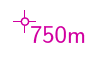from Thomas Holder for version 5.2.x…

This label requires to specify the position of text relative to point with help of -altitude. In this case -altitude bottom-right

  def p_altitude(expr pos)=
T:=identity shifted pos;
pickup PenD;
p:=(-.3u,0)--(.3u,0);
thdraw p; thdraw p rotated 90;
p:=fullcircle scaled .2u;
thclean p; thdraw p;
enddef;

vardef p_label@#(expr txt,pos,rot,mode) =
if mode=1:
thdrawoptions(withcolor .8red + .4blue);
p_altitude(pos);
% append "m" to label
picture txtm;
txtm:=image(
draw txt;
interim labeloffset:=0;
label.urt(btex \thaltitude m etex, lrcorner txt);
);
% give extra offset in case of l/r/t/b alignment
pair ctmp;
ctmp:=center thelabel@#("x", (0,0));
if (xpart ctmp * ypart ctmp)=0:
interim labeloffset:=(.4u);
else: % diagonal alignment
interim labeloffset:=(.2u);
fi;
% draw label
lab:=thelabel@#(txtm, pos);
draw lab _thop_; % use color
thdrawoptions();
bboxmargin:=0.8bp;
write_circ_bbox((bbox lab) smoothed 2);
else:
if mode=7: interim labeloffset:=(u/8) fi;
lab:=thelabel@#(txt, pos);
if mode>1: pickup PenD fi;
if mode=2: process_uplabel;
elseif mode=3: process_downlabel;
elseif mode=4: process_updownlabel;
elseif mode=5: process_circledlabel;
elseif mode=6: process_boxedlabel;
elseif mode=7: process_label(pos,rot);  % station name
elseif mode=8: process_filledlabel(pos, rot);
else: process_label(pos,rot); fi;
fi;
enddef;


#### Make 'point height' have P prefix for pits and C prefix for climbs

Martin Budaj

  code mpost
verbatimtex \def\thheightpos{C}\def\thheightneg{P} etex

and use “point 0 0 height -value [+10 m]” or “point 0 0 height -value [-85 m]” in your data to get C10 or P85.

#### Conditional Printing of Text Labels

(Thomas' 'Scale Dependant Visualization' posts below show a tidier way of redefining the built in metapost, that will better inherit future changes to the default label metapost)

Examples of North arrows, scale-bars, gridlines etc

#### I want my north arrow to have a label, like "Norte Geografico"

Carlos Grohmann & Martin Budaj

  def s_northarrow_SKBB (expr rot) =
T:=identity scaled 0.7 rotated -rot;
begingroup
interim defaultscale:=1;
label(btex Norte Geografico etex, (0,-1cm));
endgroup;
thdraw (-.5cm,-1cm)--(0,1.5cm)--(.5cm,-1cm)--(0,-.5cm)--cycle;
thfill (-.5cm,-1cm)--(0,1.5cm)--(0,-.5cm)--cycle;
enddef;

#### Northarrow 1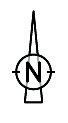from Thomas Holder for version 5.2.x…

  def s_northarrow (expr rot) =
begingroup
interim defaultscale:=0.5; % scale your north arrow here
T:=identity scaled defaultscale rotated -rot;
pickup pencircle scaled (0.08cm * defaultscale);
thdraw (-.4cm,-1.4cm)--(0,2.8cm)--(.4cm,-1.4cm)--cycle;
p:=fullcircle scaled 1.6cm;
thclean p; thdraw p;
p:=(0.95cm,0)--(0.65cm,0);
thdraw p; thdraw p xscaled -1;
pickup pencircle scaled (0.12cm * defaultscale);
p:=(0.28cm,0.42cm);
thdraw p--(p yscaled -1)--(p xscaled -1)--(p scaled -1);
endgroup;
enddef;

#### Northarrow 2from Thomas Holder for version 5.2.x…

  def s_northarrow (expr rot) =
begingroup
interim defaultscale:=0.7; % scale your north arrow here
T:=identity scaled defaultscale rotated -rot;
interim linecap:=squared;
interim linejoin:=rounded;
thfill (-.5cm,-.1cm)--(0,2.5cm)--(.5cm,-.1cm)--cycle;
pickup pencircle scaled (0.08cm * defaultscale);
thdraw (0,0)--(0,-2.5cm);
pickup pencircle scaled (0.16cm * defaultscale);
p:=(0.4cm,0.6cm);
thdraw ((p--(p yscaled -1)--(p xscaled -1)--(p scaled -1)) shifted (0,-1.0cm));
label.rt(thTEX("mg") scaled 1.6, (.6cm,-1.6cm)) transformed T;
endgroup;
enddef;


#### Northarrow 3from Stacho Mudrák for version 5.3.x…

  def s_northarrow_3 (expr rot) =
T:=identity;
picture tmp_pic;
tmp_pic = image (
begingroup
interim defaultscale:=3;
label.top("N", origin shifted (0,2.2cm));
endgroup;
thdraw (-.4cm,.4cm)--(0,2cm)--(.4cm,.4cm)--(2cm,0cm)--(.4cm,-.4cm)--(0,-2cm)--(-.4cm,-.4cm)--(-2cm,0)--cycle;
thfill (-.4cm,.4cm)--(0,2cm)--(0,0)--cycle;
thfill (.4cm,-.4cm)--(0,-2cm)--(0,0)--cycle;
thfill (.4cm,.4cm)--(2cm,0)--(0,0)--cycle;
thfill (-.4cm,-.4cm)--(-2cm,0)--(0,0)--cycle;
);
draw tmp_pic scaled 0.5 rotatedaround(origin, -rot);
enddef;

#### Northarrow 4

from Dirk Peinelt for 5.3.16…

I combine this compass rose with a creation date in the map-header (/currentdate), as a date variable is not present in metapost.

Martin Sluka: There is simple way to add actual date into Metapost code. I add modified variation after original code.

If the CS is not set, the declination is Zero, and so based on the declination, the northarrow is a Nmag arrow:

North grid/true rotate the whole plan with the north arrow. (meridianal Convergence)

def s_northarrow (expr rot) =
valscal=1.2; % scale your north arrow here
decl:=MagDecl;
T:=identity;
picture tmp_pic;
tmp_pic = image (
pickup pencircle scaled .3;
thfill fullcircle scaled 4cm withcolor 1white;
thdraw fullcircle scaled 3.1cm;
thdraw fullcircle scaled 4.05cm;
pickup pencircle scaled .1;
thdraw fullcircle scaled 3cm;
thdraw fullcircle scaled 4cm;
pickup pencircle scaled .2;
thdraw (dir(45)*2.025cm)--(dir(45)*3.7cm);
thdraw (dir(135)*2.025cm)--(dir(135)*3.7cm);
thdraw (dir(225)*2.025cm)--(dir(225)*3.7cm);
thdraw (dir(315)*2.025cm)--(dir(315)*3.7cm);
pickup pencircle scaled .1;
for whereto=0 step 15 until 345:
thdraw dir(whereto)*.65cm--dir(whereto)*.9cm;
thdraw dir(whereto)*1.4cm--dir(whereto)*1.5cm;
endfor;
for whereto=0 step 5 until 355:
thdraw dir(whereto)*.65cm--dir(whereto)*.8cm;
thdraw dir(whereto)*1.45cm--dir(whereto)*1.5cm;
endfor;
for whereto=0 step 1 until 359:
thdraw dir(whereto)*1.94cm--dir(whereto)*2cm;
endfor;
pickup pencircle scaled 1;
thdraw fullcircle scaled 1cm;
thdraw fullcircle scaled 1.1cm;
thdraw fullcircle scaled 1.3cm withpen pencircle scaled .3;
vald=90-decl;
texrot=0-decl;
drawarrow(dir(vald)*-2cm--dir(vald)*2cm) withpen pencircle scaled .2;
if (MagDecl <> 0): thdraw image(label.top(btex $mg$ etex, (0,0)) scaled .5 rotated texrot;) shifted (dir(vald)*2.04cm); fi;
thfill (1.06cm,1.06cm)--(0,.2cm)--(-1.06cm,1.06cm)--(-.2cm,0)--(-1.06cm,-1.06cm)--(0,-.2cm)--(1.06cm,-1.06cm)--(.2cm,0)--cycle;
thfill (-.2cm,.2cm)--(0,2cm)--(0,0)--cycle;
thfill (.2cm,-.2cm)--(0,-2cm)--(0,0)--cycle;
thfill (.2cm,.2cm)--(2cm,0)--(0,0)--cycle;
thfill (-.2cm,-.2cm)--(-2cm,0)--(0,0)--cycle;
thfill (.2cm,.2cm)--(-0,2cm)--(0,0)--cycle withcolor 1white;
thfill (.2cm,-.2cm)--(2cm,0)--(0,0)--cycle withcolor 1white;
thfill (-.2cm,-.2cm)--(0,-2cm)--(0,0)--cycle withcolor 1white;
thfill (-.2cm,.2cm)--(-2cm,0)--(0,0)--cycle withcolor 1white;
pickup pencircle scaled .2;
thdraw (-.2cm,.2cm)--(0,2cm)--(.2cm,.2cm)--(2cm,0cm)--(.2cm,-.2cm)--(0,-2cm)--(-.2cm,-.2cm)--(-2cm,0)--cycle;
thfill fullcircle scaled .56cm withcolor 1white;
pickup pencircle scaled .1;
thdraw (.28cm,0)..(0,.28cm)..(-.28cm,0)..(0,-.28cm)..cycle;
pickup pencircle scaled .4;
thdraw (.2cm,0)..(0,.2cm)..(-.2cm,0)..(0,-.2cm)..cycle;
if (MagDecl = 0): label.bot(btex $Nmag$ etex, (0,2.6cm)); else: label.bot(btex $N$ etex, (0,2.6cm)); fi;
label.lft(btex $E$ etex, (2.6cm,0));
label.rt(btex $W$ etex, (-2.6cm,0));
label.top(btex $S$ etex, (0,-2.6cm));
);
thdraw tmp_pic scaled valscal rotatedaround(origin, -rot);
enddef;

#### Northarrow 4a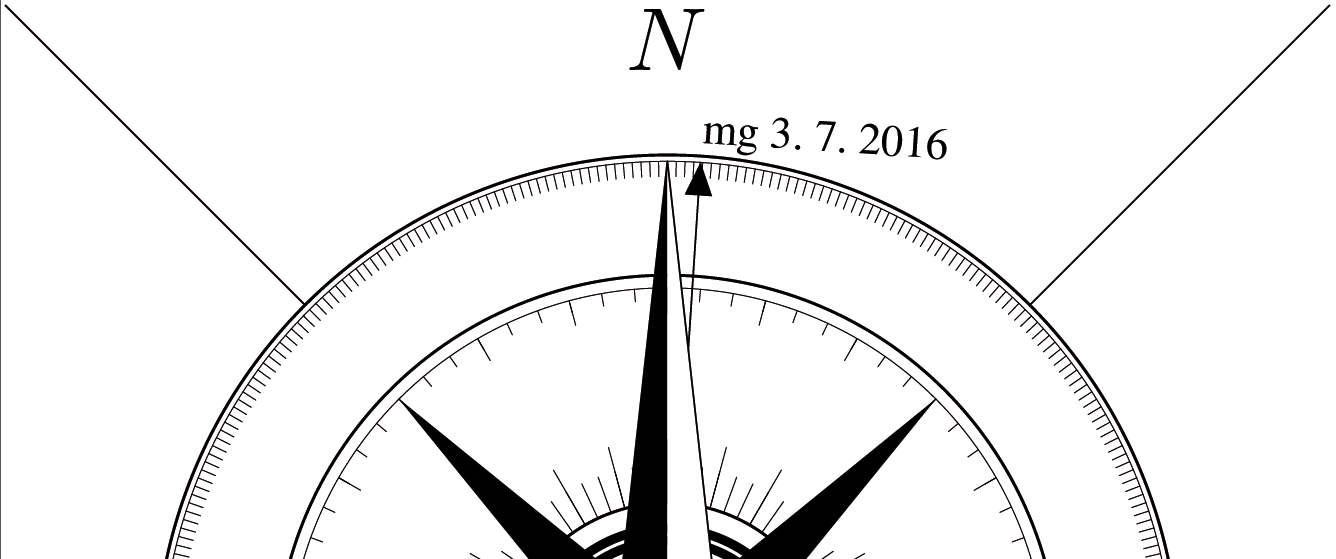With actual date
If you want to use another font, check, please, one which include character “space”.

def s_northarrow (expr rot) =
valscal=1.2; % scale your north arrow here
decl:=MagDecl;
T:=identity;
picture tmp_pic;
tmp_pic = image (
pickup pencircle scaled .3;
thfill fullcircle scaled 4cm withcolor 1white;
thdraw fullcircle scaled 3.1cm;
thdraw fullcircle scaled 4.05cm;
pickup pencircle scaled .1;
thdraw fullcircle scaled 3cm;
thdraw fullcircle scaled 4cm;
pickup pencircle scaled .2;
thdraw (dir(45)*2.025cm)--(dir(45)*3.7cm);
thdraw (dir(135)*2.025cm)--(dir(135)*3.7cm);
thdraw (dir(225)*2.025cm)--(dir(225)*3.7cm);
thdraw (dir(315)*2.025cm)--(dir(315)*3.7cm);
pickup pencircle scaled .1;
for whereto=0 step 15 until 345:
thdraw dir(whereto)*.65cm--dir(whereto)*.9cm;
thdraw dir(whereto)*1.4cm--dir(whereto)*1.5cm;
endfor;
for whereto=0 step 5 until 355:
thdraw dir(whereto)*.65cm--dir(whereto)*.8cm;
thdraw dir(whereto)*1.45cm--dir(whereto)*1.5cm;
endfor;
for whereto=0 step 1 until 359:
thdraw dir(whereto)*1.94cm--dir(whereto)*2cm;
endfor;
pickup pencircle scaled 1;
thdraw fullcircle scaled 1cm;
thdraw fullcircle scaled 1.1cm;
thdraw fullcircle scaled 1.3cm withpen pencircle scaled .3;
vald=90-decl;
texrot=0-decl;
drawarrow(dir(vald)*-2cm--dir(vald)*2cm) withpen pencircle scaled .2;
if (MagDecl <> 0):
string z;
z = ("mg " & (decimal day) & ". " & (decimal month) & ". " & (decimal year));
thdraw image(label.urt( z infont "ptmr8r", (0,0)) scaled .5 rotated texrot;) shifted (dir(vald)*2.04cm);
fi;
thfill (1.06cm,1.06cm)--(0,.2cm)--(-1.06cm,1.06cm)--(-.2cm,0)--(-1.06cm,-1.06cm)--(0,-.2cm)--(1.06cm,-1.06cm)--(.2cm,0)--cycle;
thfill (-.2cm,.2cm)--(0,2cm)--(0,0)--cycle;
thfill (.2cm,-.2cm)--(0,-2cm)--(0,0)--cycle;
thfill (.2cm,.2cm)--(2cm,0)--(0,0)--cycle;
thfill (-.2cm,-.2cm)--(-2cm,0)--(0,0)--cycle;
thfill (.2cm,.2cm)--(-0,2cm)--(0,0)--cycle withcolor 1white;
thfill (.2cm,-.2cm)--(2cm,0)--(0,0)--cycle withcolor 1white;
thfill (-.2cm,-.2cm)--(0,-2cm)--(0,0)--cycle withcolor 1white;
thfill (-.2cm,.2cm)--(-2cm,0)--(0,0)--cycle withcolor 1white;
pickup pencircle scaled .2;
thdraw (-.2cm,.2cm)--(0,2cm)--(.2cm,.2cm)--(2cm,0cm)--(.2cm,-.2cm)--(0,-2cm)--(-.2cm,-.2cm)--(-2cm,0)--cycle;
thfill fullcircle scaled .56cm withcolor 1white;
pickup pencircle scaled .1;
thdraw (.28cm,0)..(0,.28cm)..(-.28cm,0)..(0,-.28cm)..cycle;
pickup pencircle scaled .4;
thdraw (.2cm,0)..(0,.2cm)..(-.2cm,0)..(0,-.2cm)..cycle;
if (MagDecl = 0): label.bot(btex $Nmag$ etex, (0,2.6cm)); else: label.bot(btex $N$ etex, (0,2.6cm)); fi;
label.lft(btex $E$ etex, (2.6cm,0));
label.rt(btex $W$ etex, (-2.6cm,0));
label.top(btex $S$ etex, (0,-2.6cm));
);
thdraw tmp_pic scaled valscal rotatedaround(origin, -rot);
enddef;

#### Scalebar 1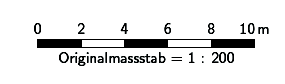from Thomas Holder for version 5.2.x…

  def s_scalebar (expr l, units, txt) =
begingroup
interim warningcheck:=0;
tmpl:=l / Scale * cm * units / 2;
tmpx:=l / Scale * cm * units / 5;
tmph:=5bp; % bar height
endgroup;
pickup PenC;
draw (-tmpl,0)--(tmpl,0)--(tmpl,-tmph)--(-tmpl,-tmph)--cycle;
p:=(0,0)--(tmpx,0)--(tmpx,-tmph)--(0,-tmph)--cycle;
for i:=-2.5 step 2 until 2:
fill p shifted (i * tmpx,0);
endfor;
begingroup
interim labeloffset:=3.5bp;
for i:=0 step (l/5) until (l-1):
tmpx:=tmpl * (i * 2 / l - 1);
label.top(thTEX(decimal (i)),(tmpx,0));
endfor;
label.top(thTEX(decimal (l) & "\thinspace" & txt),(tmpl,0));
label.bot(thTEX("Originalmassstab = 1 : " & decimal round(Scale*100)),(0,-tmph));
endgroup;
enddef;

#### Scalebar 2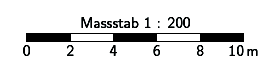from Thomas Holder for version 5.2.x…

  def s_scalebar (expr l, units, txt) =
begingroup
interim warningcheck:=0;
tmpl:=l / Scale * cm * units / 2;
tmpx:=l / Scale * cm * units / 5;
tmph:=5bp; % bar height
endgroup;
pickup PenC;
draw (-tmpl,0)--(tmpl,0)--(tmpl,-tmph)--(-tmpl,-tmph)--cycle;
p:=(0,0)--(tmpx,0)--(tmpx,-tmph)--(0,-tmph)--cycle;
for i:=-2.5 step 2 until 2:
fill p shifted (i * tmpx,0);
endfor;
begingroup
interim labeloffset:=3.5bp;
for i:=0 step (l/5) until (l-1):
tmpx:=tmpl * (i * 2 / l - 1);
label.bot(thTEX(decimal (i)),(tmpx,-tmph));
endfor;
label.bot(thTEX(decimal (l) & "\thinspace" & txt),(tmpl,-tmph));
label.top(thTEX("Massstab 1 : " & decimal round(Scale*100)),(0,0));
endgroup;
enddef;

from Andrew Atkinson

Some of us have fussy editors, who point out that due to the drawing of the rectangle for the scale bar the black lines are not exactly the same size as the white ones.

To fix this for the two scale bars above

Replace

  draw (-tmpl,0)--(tmpl,0)--(tmpl,-tmph)--(-tmpl,-tmph)--cycle;


with

  #Adjusted to make lines not stick out: 0.25*u/10,0 is half thickness of PenC
draw (-tmpl+0.25*u/10,0)--(tmpl-0.25*u/10,0);
draw (tmpl-0.25*u/10,-tmph)--(-tmpl+0.25*u/10,-tmph);

#### Change grid symbols from cross hairs to continuous lines

from Stacho Mudrak (mostly) and Bruce Mutton 2007

  code metapost
def s_hgrid (expr xpos, ypos, xsize, ysize) =
pickup PenD;
draw (
if xpos < 0: 0 else: -xsize/2 fi, 0
) -- (
if xpos > 0: 0 else: xsize/2 fi, 0
) withcolor 0.1black+0.5white;
draw (
0, if ypos < 0: 0 else: -ysize/2 fi
) -- (
0, if ypos > 0: 0 else: ysize/2 fi
) withcolor 0.1black+0.5white;
enddef;
endcode

Examples of scale dependent labeling and symbol display etc

A lot of this does not require metapost code (ie much of it can be done with default therion layout syntax), so maybe put this topic on a page of it's own?

from Thomas Holder for version 5.2.x…

  def l_rockborder (expr p) =
if abs(llcorner p - urcorner p) > u:
l_rockborder_UIS(p);
fi;
enddef;

def l_rockedge (expr p) =
if abs(llcorner p - urcorner p) > u:
l_rockedge_UIS(p);
fi;
enddef;

Examples of symbols that plot differently, dependant on whether particular colour variables are defined. Provides an easy way to toggle between producing maps optimised for black and white, and maps optimised for colour production.

Code to redefine area and point water symbols
Bruce Mutton 2010-2011 for Therion 5.3.8, 5.3.9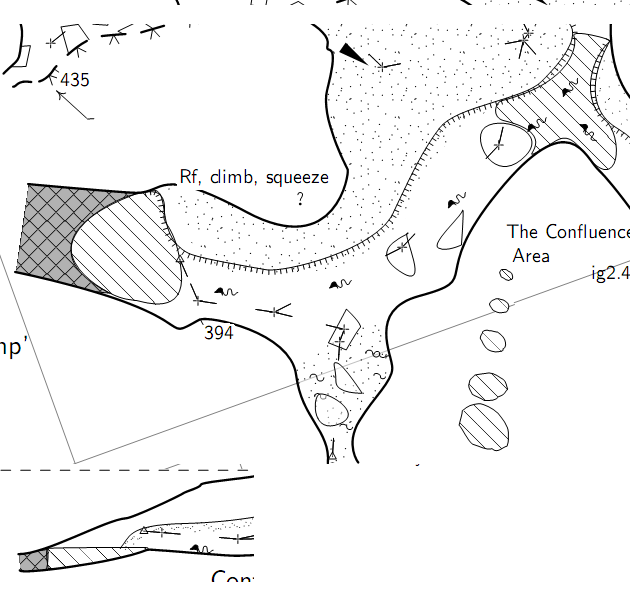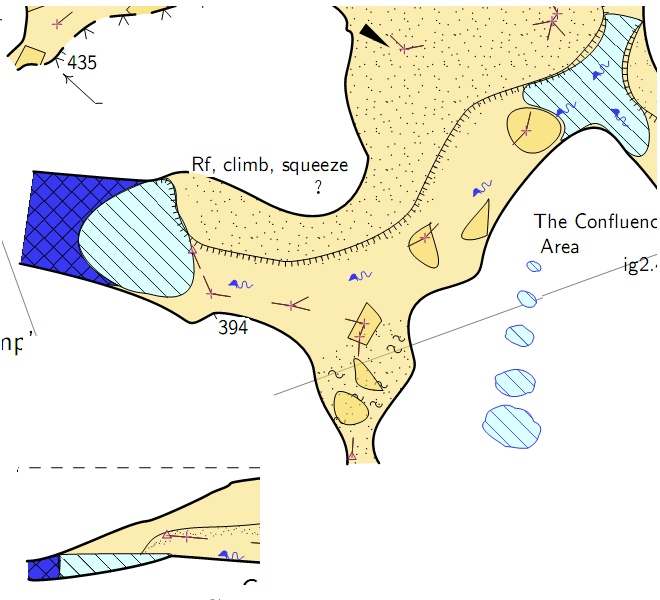The area water symbols have much more spaced hatch lines, more consistent with what we traditionally use in New Zealand. The black and white point and area water has no background shading whereas the colour versions do.

  #Patterns similar to standard definition of pattern_water_UIS and pattern_sump_UIS
#Gives b&w shaded symbols with borders, unless custom colours are defined
#in which case colour backgrounds defined in LayoutColourSymbols are used
code metapost
beginpattern(pattern_water);
draw origin--10up withpen pensquare scaled (0.04u); % line thickness
patternxstep(.50u);                                 % spacing
patterntransform(identity rotated 45);
endpattern;

beginpattern(pattern_sump);
draw origin--(0,.50u) withpen pensquare scaled (0.04u);
draw origin--(.50u,0) withpen pensquare scaled (0.04u);
patterntransform(identity rotated 45);
endpattern;

def p_water (expr pos,theta,sc,al)=
%Bruce Mutton 2010.06.20 for Therion 5.3.8
U:=(.425u,.3u);
T:=identity aligned al rotated theta scaled sc shifted pos;
pickup PenD;
p:=fullcircle xscaled (1.2u) yscaled (0.8u) randomized (0.1u) rotated uniformdeviate (-45);
if known colour_water_bg: thfill p withcolor colour_water_bg; else: thfill p withcolor white; fi;
thfill p withpattern pattern_water; %%% withcolor black;  %but with failed attempt at black hatch
thdraw p;   %outline
enddef;

def a_water (expr Path) =
%Bruce Mutton 2010.06.20 for Therion 5.3.8
T:=identity;
thclean Path;
pickup PenD;
if known colour_water_bg: thfill Path withcolor colour_water_bg; else: thfill Path withcolor white; fi;
thfill Path withpattern pattern_water;
%%thdraw Path;  %outline, not needed as area drawn is defined by line border anyway
%and border can be made invisible with subtype invisible where water meets sump in elevation
enddef;

def a_sump (expr Path) =
%Bruce Mutton 2010.06.20 for Therion 5.3.8
T:=identity;
thclean Path;
pickup PenD;
if known colour_sump_bg:  thfill Path withcolor colour_sump_bg; else: thfill Path withcolor 0.7white; fi;
thfill Path withpattern pattern_sump;
%%thdraw Path;  %outline, not needed as area drawn is defined by line border anyway
%and border can be made invisible with subtype invisible where water meets sump in elevation
enddef;
endcode

Code to define custom line rope symbol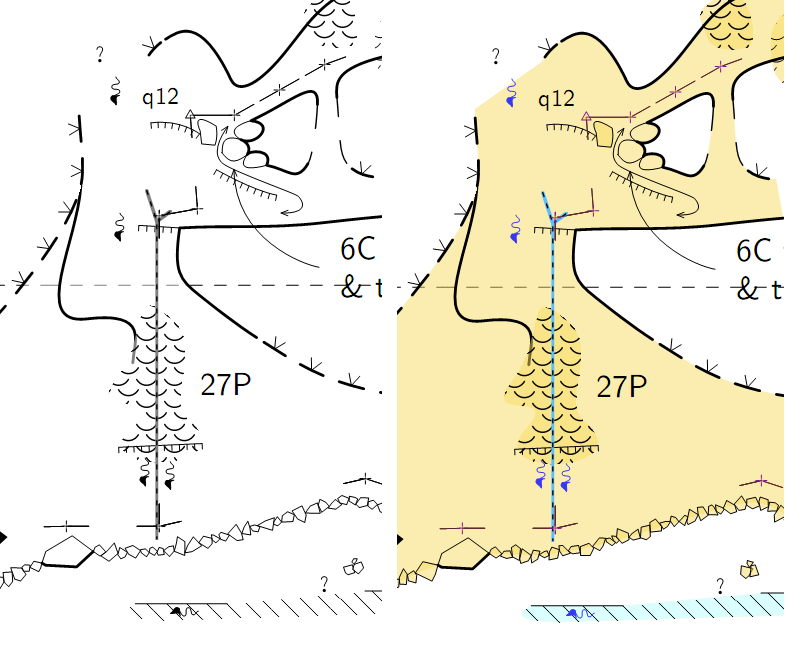code metapost
#submitted by Christian Jackson Nov2011
#modified for colour or black and white Bruce Mutton Nov2011
def l_u_rope (expr P) =
T:=identity;
pickup pensquare scaled (1.0*u/10);
if known colour_rope: thdraw P withcolor colour_rope; else: thdraw P withcolor 0.1black+0.5white;
fi;
pickup PenC;
thdraw P dashed dashpattern(on 1bp off 2bp on 1bp off 2bp);
enddef;

initsymbol("l_u_rope")

def l_u_rope_legend =
l_u_rope(((.2,.2) -- (.8,.8)) inscale)
enddef;
endcode

Need to add next line to all thconfig files to define legend text for the new custom rope symbol

  text en "line u:rope" "rope" #text to appear in legend

Code to make full colour symbols
Here is a layout where you can define the colours you would like to use with the above code when you don't want a black and white map. (The groups of the statements in the 'code metapost' block are the ones that work with the code above).
I have not included any opacity statements in here, but perhaps it would be a good idea. The degree of opacity that works well for black and white outputs seems to be quite different to that which works for colour outputs.


layout LayoutColourSymbols
#Still experimental (Dec 2011) and as per the comments in the code below, many of the default
#'symbol-colour' statements do not work as I would like.  The custom metapost
#components work fine however.

#affects colour symbol linework but not labels and not fills

symbol-colour point station [54 7 60]	#purple[54 7 60] #seems to affect +s, flag symbols and station text

#survey cave
symbol-colour group cave-centreline [35 16 16] # brown [35 16 16] # red brown [50 0 0]
symbol-colour point cave-station [35 16 16]  #ineffective?
#symbol-colour point station-name [0 100 0]

#survey surface
symbol-colour group surface-centreline [54 74 29] # green [54 74 29]
symbol-colour point surface-station [54 74 29]  #ineffective?
symbol-colour point flag:entrance [54 74 29]  #ineffective?

#magenta
symbol-colour group equipment  [80 0 40] # magenta [80 0 40]

#dark blue
# symbol-colour group water  [22 22 95] # this breaks custom water definitions
symbol-colour point water-flow  [22 22 95]
symbol-colour line water-flow  [22 22 95]
symbol-colour point water [22 22 95]	    # inconsistent?
# symbol-colour area sump [22 22 95]	# this breaks custom water definitions

#light blue
# symbol-colour area water [86 100 100]	# this breaks custom water definitions

#define colours for redefined water point & area
code metapost
%these colours affect fills, not the linework
!color colour_water_bg; %! forces interpretation as metapost
colour_water_bg := (0.86,1,1);      %light blue
!color colour_sump_bg;  %! forces interpretation as metapost
colour_sump_bg := (.22,.22,.95);    %dark blue

%these colours affect the linework
!color colour_rope;  %! forces interpretation as metapost
colour_rope :=  (0.35,0.75,1.0);    %blue

%this colour for entrance symbol (not point station flag entrance)
!color colour_entrance;  %! forces interpretation as metapost
colour_entrance :=  (0.54,0.74,0.29);
endcode
endlayout LayoutColourSymbols

And the 'switch' that allows you to easily toggle from black and white to colour…
Just include this line in your thconfig file (or a layout referenced from the thconfig.

   copy LayoutColourSymbols #excludes labels


Comment it out when you want black and white.

• metapost.1559511302.txt.gz